# FALCOR

Fluctuation AnaLysis CalculatOR
The program FALCOR is a web tool designed for use with Luria-Delbrück fluctuation analysis to calculate the mutation frequency and rate from various mutation assays in bacteria and yeast (e.g. resistance to canavanine or erythromycin, reversion to Trp+, etc.). Three calculation methods are available through this program: (1) Ma-Sandri-Sarkar Maximum Likelihood Estimator (MSS-MLE) Method, (2) Lea-Coulson Method of the Median (LC) and (3) Frequency. It is assumed that any potential user of FALCOR has an understanding of fluctuation analysis, and how to conduct experiments properly. Foster (2006) provides an excellent review of fluctuation analysis, including the underlying assumptions that shape the current mathematical models. Information regarding the user interface and statistical calculations implemented by FALCOR can be found below.

BACKGROUND:

Originally described by Luria & Delbrück (1943), fluctuation analysis has become the standard method in the field for calculating mutation rates. Briefly, a small number of cells are used to inoculate parallel cultures in a non-selective medium. The cultures are then grown to saturation to obtain equal cell densities. Cells are then plated onto selective media to obtain the number of mutants, r, and dilutions are plated onto rich medium to calculate the total number of viable cells, Nt. Frequency is not a sufficiently accurate measure of mutation; mutation rate should always be calculated (Rosche and Foster, 2000; Schmidt et al., 2006).

A number of statistical methods have been developed to estimate the number of mutations, m, from the observed values of mutants, r, across parallel cultures. While the Lea-Coulson method of the median (LC), introduced in 1949, is the classic model for the estimation of mutation rates, statistical analyses have evolved to more accurately estimate m. However, the complex calculations required place these more accurate methods beyond easy reach of bench scientists. The Ma-Sandri-Sarkar Maximum Likelihood Estimator (MSS-MLE) is the best method available to date; it is the most accurate and, unlike the LC method, is valid over all values of r and m. Furthermore, the MSS-MLE method calculates the mutation rate from the entire data set (not just the median), providing more statistical power. A comprehensive evaluation of these methods was conducted with experimental data by Rosche and Foster (2000). To facilitate the use of these complicated methods by bench scientists, we developed a web interface to implement these three most popular methods.

INTERFACE:

Input

Values of 'r' represent the number of mutants (or revertants) on selective media plates. Values of 'N' represent the total number of cells plated onto the selective media. To calculate 'N', diluted cultures are plated onto rich medium. Values of 'r' and 'N' have to be normalized to the same volume (such as 1 mL). As such, you obtain 'r' mutants when 1 mL of an overnight culture is plated on selective media, with 1 mL of media containing 'N' number of cells. It is recommended that the user input data from Excel into the '2 column entry' input box, with column 1 = r, column 2 = N. However, values can be entered directly into the r and N input boxes. A sample data set is provided along with corresponding output for the various methods of analysis. Click here for data set

Grouping

Multiple data can be entered into the program at the same time, and grouped together (as specified by the user) for various output. For example, an experiment with 10 cultures is repeated 3 times. Grouping by 30 gives the median and confidence interval across all data points. Grouping by 10 gives the medians and confidence intervals for each of the 3 experiments.

Rate Output

The magnitude of the output can be controlled by the user by entering which log value of 10 to express the rate (default value is 10^-7). "Combine rate output" is designed to make it easier for creation of excel graphs. The rate and confidence interval range and difference about the median are combined into one text field for easier output handling. The confidence interval difference should be used to make error bars in Excel.

IMPLEMENTATION:

MSS-Maximum Likelihood Estimator Method (MSS-MLE)
While the Lea-Coulson Method of the Median is the most commonly used in the literature, the MSS-Maximum Likelihood Method is currently the best method to estimate m. The MSS-MLE method uses an initial estimate of m to generate the probability of observing r mutants on selective medium, pr (Eq. 1). The likelihood function is the product of the pr’s for each observed value of r (Eq. 2). The value of m is then adjusted until the likelihood function reaches a maximum (Sarkar et al., 1992; Ma et al., 1992). The mutation rate, M, is then defined as m/Ñt, where Ñt represents the average of the cell counts across the cultures. As such, FALCOR first normalizes all values of r to Ñt, as determined by how the data is grouped. The confidence intervals are calculated according to the method of Stewart (1994) who discovered that the natural log of m is normally distributed. The confidence intervals calculated by FALCOR are derived from an approximation of this distribution (Eq. 3), as described by Rosche and Foster (2000).

Eq. 1: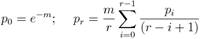Eq. 2: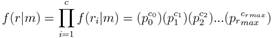Eq. 3: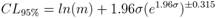; where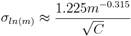Lea-Coulson Method of the Median
FALCOR implements a slightly modified version of the LC method (Schmidt et al. 2006). Briefly, the value of m is calculated from r via the Lea-Coulson equation (Eq. 4). The mutation rate, M, is then determined for each data point: m/Nt. The program then sorts the values of M and determines the median. This method performs well over the range 2.5 < r < 60 (1.5 < m < 15). Confidence intervals are derived from the cumulative binomial distribution of the rank-values (Rosche and Foster, 2000). The probability mass function of a binomial distribution used by FALCOR is given in Eq. 5.

Eq. 4: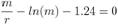Eq. 5: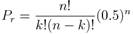Frequency
This method determines the frequency of mutation (i.e., r/N). However, frequency is highly inaccurate, and in cases of measuring spontaneous mutations, rates should be calculated to obtain a accurate representation of the data. Frequencies are useful for determining the level of induced mutations in an population. FALCOR calculates frequency, providing statistical interpretation of the data with confidence intervals about the median, as with the LC method (based on Eq. 5).

REFERENCES:

Foster,P.L. (2006) Methods for Determining Spontaneous Mutation Rates.  Methods Enzymol., 409, 195-213.

Lea,D.E. and Coulson,C.A. (1949) The distribution of the numbers of mutants in bacterial populations. J. Genet. 49: 264-285.

Luria,S.E. and Delbrück,M. (1943) Mutations of bacteria from virus sensitivity to virus resistance. Genetics, 28: 491-511.

Ma,W.T., Sandri,G.v.H. and Sarkar,S. (1992). Analysis of the Luria-Delbrück distribution using discrete convolution powers. J Appl Prob, 29: 255-267.

Rosche,W.A. and Foster,P.L. (2000) Determining Mutation Rates in Bacterial Populations. Methods, 20, 4-17.

Sarkar,S., Ma,W.T. and Sandri,G.v.H. (1992) On fluctuation analysis: a new, simple and efficient method for computing the expected number of mutants. Genetica, 85: 173-179.

Schmidt,K.H., Pennaneach,V., Putnam,C.D. and Kolodner,R.D. (2006) Chapter 27: Analysis of Gross-Chromosomal Rearrangements in Saccharomyces cerevisiae. Methods Enzymol., 409: 462-476.

Stewart,F.M. (1994) Fluctuation tests: how reliable are the estimates of mutation rates? Genetics, 137: 1139-1146.

CITATION:

Hall BM, Ma CX, Liang P, Singh KK. Fluctuation analysis CalculatOR: a web tool for the determination of mutation rate using Luria-Delbruck fluctuation analysis. Bioinformatics. 2009;25(12):1564-1565. link to the paper

Papers citing this tool can be found here

CURRENT CONTACT INFORMATION:

Ping Liang, PhD, Professor
Department of Biological Sciences
Brock University
St. Catharines, Ontario